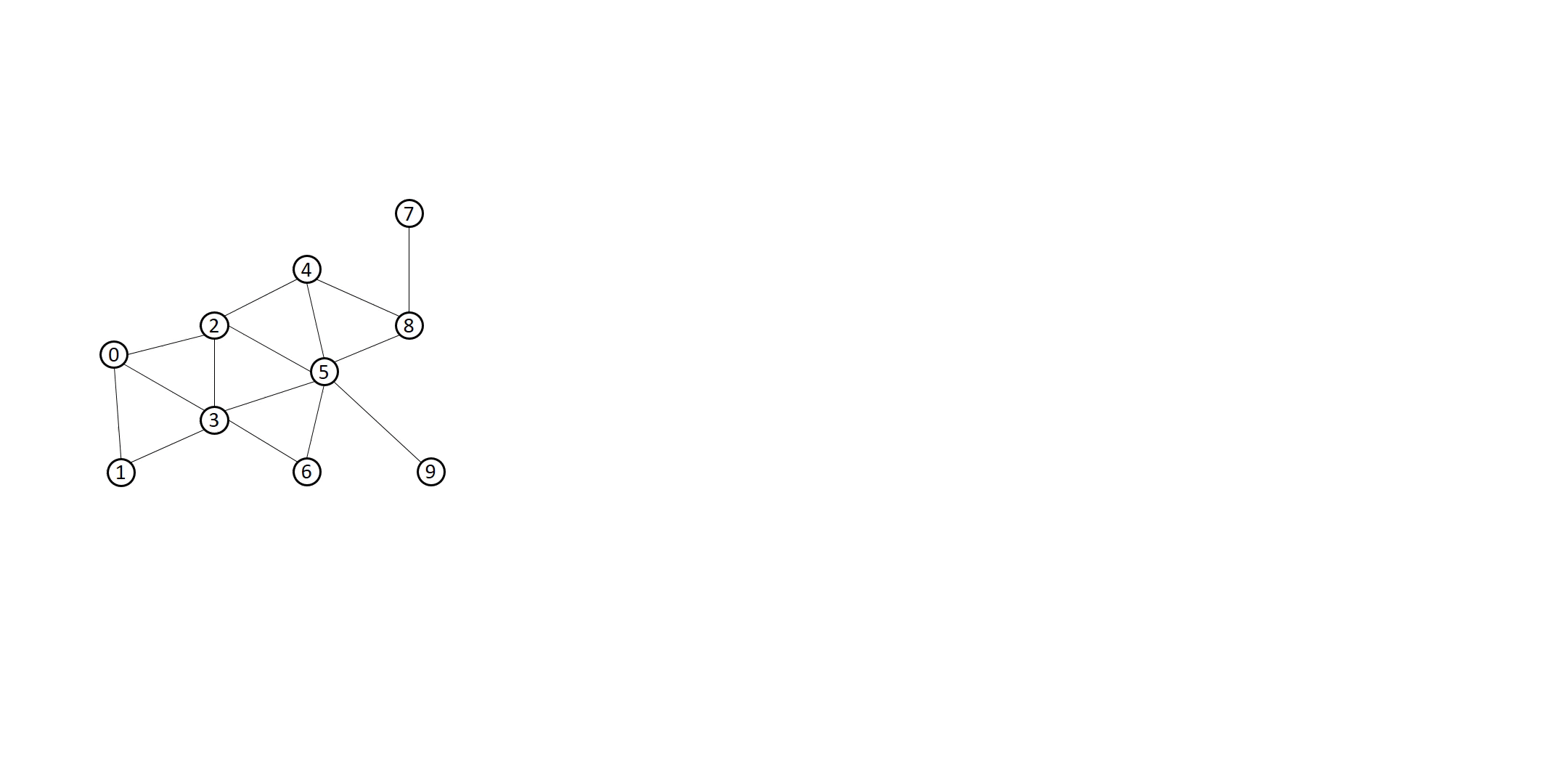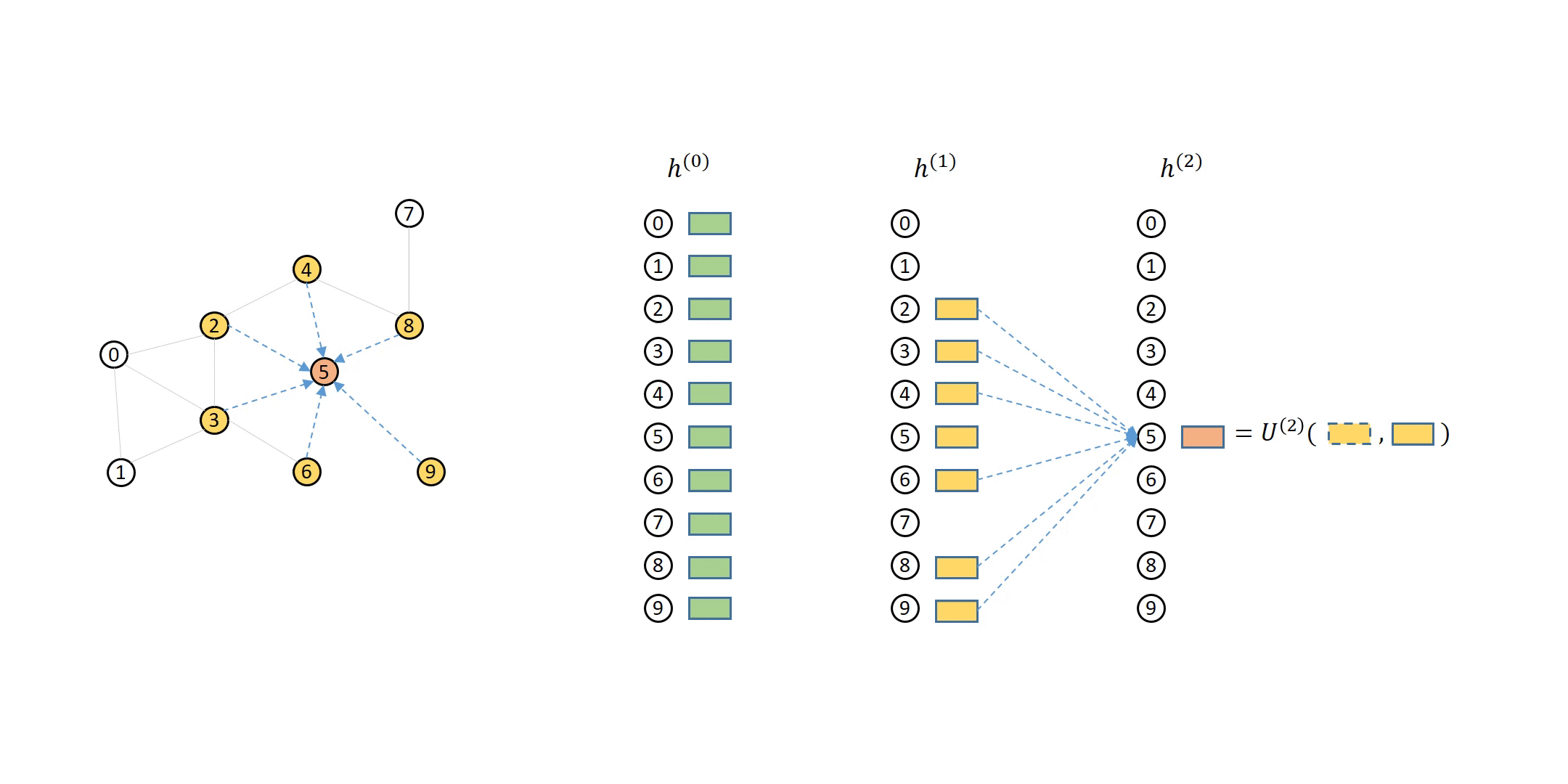# Introduction of Neighbor Sampling for GNN Training¶

In previous tutorials you have learned how to train GNNs by computing the representations of all nodes on a graph. However, sometimes your graph is too large to fit the computation of all nodes in a single GPU.

By the end of this tutorial, you will be able to

• Understand the pipeline of stochastic GNN training.

• Understand what is neighbor sampling and why it yields a bipartite graph for each GNN layer.

## Message Passing Review¶

Recall that in Gilmer et al. (also in message passing tutorial), the message passing formulation is as follows:

$m_{u\to v}^{(l)} = M^{(l)}\left(h_v^{(l-1)}, h_u^{(l-1)}, e_{u\to v}^{(l-1)}\right)$
$m_{v}^{(l)} = \sum_{u\in\mathcal{N}(v)}m_{u\to v}^{(l)}$
$h_v^{(l)} = U^{(l)}\left(h_v^{(l-1)}, m_v^{(l)}\right)$

where DGL calls $$M^{(l)}$$ the message function, $$\sum$$ the reduce function and $$U^{(l)}$$ the update function. Note that $$\sum$$ here can represent any function and is not necessarily a summation.

Essentially, the $$l$$-th layer representation of a single node depends on the $$(l-1)$$-th layer representation of the same node, as well as the $$(l-1)$$-th layer representation of the neighboring nodes. Those $$(l-1)$$-th layer representations then depend on the $$(l-2)$$-th layer representation of those nodes, as well as their neighbors.

The following animation shows how a 2-layer GNN is supposed to compute the output of node 5:You can see that to compute node 5 from the second layer, you will need its direct neighbors’ first layer representations (colored in yellow), which in turn needs their direct neighbors’ (i.e. node 5’s second-hop neighbors’) representations (colored in green).

## Neighbor Sampling Overview¶

You can also see from the previous example that computing representation for a small number of nodes often requires input features of a significantly larger number of nodes. Taking all neighbors for message aggregation is often too costly since the nodes needed for input features would easily cover a large portion of the graph, especially for real-world graphs which are often scale-free.

Neighbor sampling addresses this issue by selecting a subset of the neighbors to perform aggregation. For instance, to compute $$\boldsymbol{h}_5^{(2)}$$, you can choose two of the neighbors instead of all of them to aggregate, as in the following animation:You can see that this method uses much fewer nodes needed in message passing for a single minibatch.

You can also notice in the animation above that the computation dependencies in the animation above can be described as a series of bipartite graphs. The output nodes (called destination nodes) are on one side and all the nodes necessary for inputs (called source nodes) are on the other side. The arrows indicate how the sampled neighbors propagates messages to the nodes. DGL calls such graphs message flow graphs (MFG).

Note that some GNN modules, such as SAGEConv, need to use the destination nodes’ features on the previous layer to compute the outputs. Without loss of generality, DGL always includes the destination nodes themselves in the source nodes.

## What’s next?¶

Stochastic GNN Training for Node Classification in DGL

# Thumbnail credits: Understanding graph embedding methods and their applications, Mengjia Xu
# sphinx_gallery_thumbnail_path = '_static/large_L0_neighbor_sampling_overview.png'


Total running time of the script: ( 0 minutes 0.000 seconds)

Gallery generated by Sphinx-Gallery